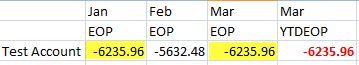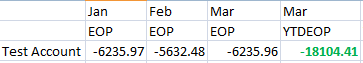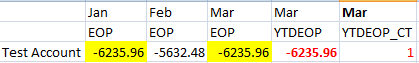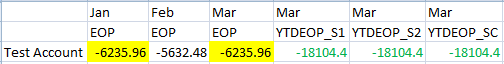# When case is not the case in an Essbase ASO cube

## Identical values in one Essbase ASO cube field lead to incorrect calculations in another. Find out what the problem is and how to fix it.

In one of our projects, we recently ran into an issue where an existing Year-To-Date member calculation in an Essbase...

ASO cube did not return the correct results for some accounts. After some detailed investigations, we realized the issue had to do with the EndOfPeriod (EOP) members.

First, we realized that the issue only occurred when there were two or more EOP members with identical values, like in the example below.Jan and Mar EOP values are identical, and as a result, the YTDEOP calculation didn't calculate correctly in the Essbase ASO cube. We changed one of the EOP values slightly so that all EOP values are unique, and the YTDEOP calculated correctly.The Multidimensional Expressions (MDX) formula written to calculate YTDEOP was a simple CASE statement, like the example below.

```CASE [Period].CurrentMember
when[JAN] then ([JAN],[EOP])
when[FEB] then ([JAN],[EOP]) + ([FEB],[EOP])
when[MAR] then ([JAN],[EOP]) + ([FEB],[EOP]) + ([MAR],[EOP])
…
```

This looked like a perfectly fine calculation and it did calculate correctly in most circumstances. It was only when two or more EOP values were identical in the Essbase ASO cube that it didn't calculate as expected. To investigate how the calculation really behaved, we created a new calculated member (YTDEOP_CT) with a simple CASE statement like in the example below.

```CASE [Period].CurrentMember

WHEN[JAN] then 1
WHEN[FEB] then 2
WHEN[MAR] then 3
…
```

And lo and behold, the returned number was 1, not 3, for Mar YTDEOP_CT.This indicates that the simple CASE statement in the calculation does not compare with the member name, but with the value of the member. When it gets to the March member, the value is the same as what is in Jan, so it does the calculation at the January member, which in the example is just that value. Basically the value -6235.96 is the same as the value in Jan, so therefore it meets a "true" condition in the CASE statement and the result is Jan, EOP.

The correct CASE statement in this case, no pun intended, is to do a Searched Case statement that references the member name and not the value.

```CASE

WHEN IS ([Period].CurrentMember, [JAN]) THEN ([JAN], [EOP])
WHEN IS ([Period].CurrentMember,  [FEB])  then ([JAN],[EOP]) + ([FEB],[EOP])
WHEN IS ([Period].CurrentMember,  [MAR]) then ([JAN],[EOP]) + ([FEB],[EOP]) + ([MAR],[EOP])
…
```

Or, better yet, don't use a CASE statement in the Essbase ASO cube at all. Instead, write an MDX calculation like one of the examples below. One line of MDX code, fully dynamic, is easier to maintain and has less of a chance for typos or copy-paste errors.

```YTDEOP_S1:
SUM([JAN]:[Period].CurrentMember, [EOP])
OR  YTDEOP_S2:
SUM(PeriodsToDate(), [EOP])
```

Note that the PeriodsToDate function requires that the Period dimension is tagged as a Time dimension.